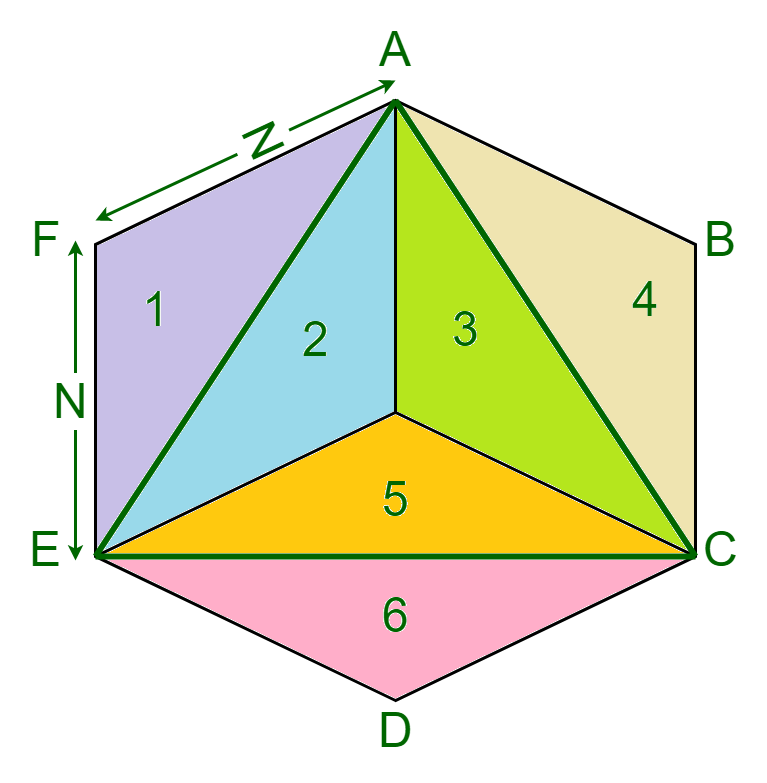Related Articles
Program to find Area of Triangle inscribed in N-sided Regular Polygon
• Last Updated : 16 Mar, 2021

Given the triangle inscribed in an N-sided regular polygon with given side length, formed using any 3 vertices of the polygon, the task is to find the area of this triangle.
Examples:

```Input: N = 6, side = 10
Output: 129.904

Input: N = 8, side = 5
Output: 45.2665```

Approach: Consider the 1st example:

• Given is a 6 sided regular polygon ABCDEF with a triangle AEC inscribed in it.

• As it can be seen, the triangle divides given polygon into 6 equal triangular areas, where the point of intersection of triangle AEC is the centroid of the triangle.•

• Area of each of the triangulated part will be (TriangulatedArea = Area of N sided regular polygon / N) from the law of symmetry.

• Since the Triangle ACE comprises of 3 out of 6 in it, So the area of triangle ACE will be (3 * TriangulatedArea)

• Therefore, in general, if there is an N-sided regular polygon with area A, the area of a triangle inscribed in it will be (A/N)*3.

Below is the implementation of the above approach:

## C++

 `// C++ Program to find the area of a triangle``// inscribed in N-sided regular polygon` `#include ``#include ``using` `namespace` `std;` `// Function to find the area of the polygon``double` `area_of_regular_polygon(``double` `n, ``double` `len)``{` `    ``// area of a regular polygon with N sides``    ``// and side length len``    ``double` `P = (len * n);``    ``double` `A``        ``= len``          ``/ (2 * ``tan``((180 / n)``                     ``* 3.14159 / 180));``    ``double` `area = (P * A) / 2;` `    ``return` `area;``}` `// Function to find the area of a triangle``double` `area_of_triangle_inscribed(``double` `n, ``double` `len)``{` `    ``double` `area = area_of_regular_polygon(n, len);` `    ``// area of one triangle``    ``// in an N-sided regular polygon``    ``double` `triangle = area / n;` `    ``// area of inscribed triangle``    ``double` `ins_tri = (triangle * 3);` `    ``return` `ins_tri;``}` `// Driver code``int` `main()``{``    ``double` `n = 6, len = 10;` `    ``cout << area_of_triangle_inscribed(n, len)``         ``<< endl;` `    ``return` `0;``}`

## Java

 `// Java Program to find the area of a triangle``// inscribed in N-sided regular polygon``import` `java.util.*;` `class` `GFG``{` `// Function to find the area of the polygon``static` `double` `area_of_regular_polygon(``double` `n,``                                      ``double` `len)``{` `    ``// area of a regular polygon with N sides``    ``// and side length len``    ``double` `P = (len * n);``    ``double` `A = len / (``2` `* Math.tan((``180` `/ n) *``                             ``3.14159` `/ ``180``));``    ``double` `area = (P * A) / ``2``;` `    ``return` `area;``}` `// Function to find the area of a triangle``static` `double` `area_of_triangle_inscribed(``double` `n,``                                         ``double` `len)``{``    ``double` `area = area_of_regular_polygon(n, len);` `    ``// area of one triangle``    ``// in an N-sided regular polygon``    ``double` `triangle = area / n;` `    ``// area of inscribed triangle``    ``double` `ins_tri = (triangle * ``3``);` `    ``return` `ins_tri;``}` `// Driver code``static` `public` `void` `main(String[] arg)``{``    ``double` `n = ``6``, len = ``10``;` `    ``System.out.printf(``"%.3f"``,``           ``area_of_triangle_inscribed(n, len));``}``}` `// This code is contributed by PrinciRaj1992`

## Python3

 `# Python3 Program to find the area``# of a triangle inscribed in``# N-sided regular polygon``import` `math` `# Function to find the area of the polygon``def` `area_of_regular_polygon(n, ``len``):` `    ``# area of a regular polygon with``    ``# N sides and side length len``    ``P ``=` `(``len` `*` `n);``    ``A ``=` `len` `/` `(``2` `*` `math.tan((``180` `/` `n) ``*``                      ``3.14159` `/` `180``))``    ``area ``=` `(P ``*` `A) ``/` `2` `    ``return` `area` `# Function to find the area of a triangle``def` `area_of_triangle_inscribed(n, ``len``):` `    ``area ``=` `area_of_regular_polygon(n, ``len``)` `    ``# area of one triangle``    ``# in an N-sided regular polygon``    ``triangle ``=` `area ``/` `n` `    ``# area of inscribed triangle``    ``ins_tri ``=` `(triangle ``*` `3``);` `    ``return` `ins_tri` `# Driver code``n ``=` `6``len` `=` `10``print``(``round``(area_of_triangle_inscribed(n, ``len``), ``3``))` `# This code is contributed by divyamohan`

## C#

 `// C# Program to find the area of a triangle``// inscribed in N-sided regular polygon``using` `System;``                    ` `class` `GFG``{` `// Function to find the area of the polygon``static` `double` `area_of_regular_polygon(``double` `n,``                                      ``double` `len)``{` `    ``// area of a regular polygon with N sides``    ``// and side length len``    ``double` `P = (len * n);``    ``double` `A = len / (2 * Math.Tan((180 / n) *``                             ``3.14159 / 180));``    ``double` `area = (P * A) / 2;` `    ``return` `area;``}` `// Function to find the area of a triangle``static` `double` `area_of_triangle_inscribed(``double` `n,``                                         ``double` `len)``{``    ``double` `area = area_of_regular_polygon(n, len);` `    ``// area of one triangle``    ``// in an N-sided regular polygon``    ``double` `triangle = area / n;` `    ``// area of inscribed triangle``    ``double` `ins_tri = (triangle * 3);` `    ``return` `ins_tri;``}` `// Driver code``static` `public` `void` `Main(String[] arg)``{``    ``double` `n = 6, len = 10;` `    ``Console.Write(``"{0:F3}"``,``            ``area_of_triangle_inscribed(n, len));``}``}` `// This code is contributed by PrinciRaj1992`

## Javascript

 ``
Output:
`129.904`

My Personal Notes arrow_drop_up